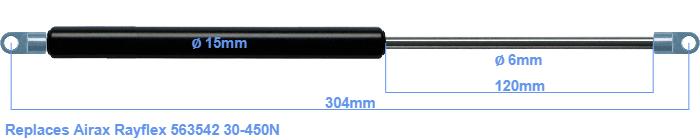# Replacement for Airax Rayflex 563542 30-450N

\$28.94

Replacement gas spring for the Airax Rayflex 563542 30-450 Newton. The eye has a thickness of 3mm and a hole diameter of 6.1mm. Brand: HAHN, a Stabilus company.

Please note:
The eye with the thickness of 3mm of this replacement gas spring can be shorter than the original. Note that this part of the eye doesn't get in the way.
 Force Choose an option30 Newton40 Newton50 Newton60 Newton80 Newton100 Newton120 Newton140 Newton150 Newton160 Newton180 Newton200 Newton220 Newton240 Newton250 Newton260 Newton280 Newton300 Newton320 Newton340 Newton350 Newton360 Newton380 Newton400 Newton420 Newton440 Newton450 NewtonClear
This gas spring is also known as 5000563542, 563542.00.This gas spring has a cylinder diameter of 15 mm. The cross section of the rod is 6 mm. The stroke of the gas spring is 120 millimeter. The total length of this replacement is exactly 304 mm. Note: this is the total length between the rotation points of the two mounting parts. The length from thread to thread (wihout mounting parts) is 272 mm. The force of this replacement gas spring is 30-450 Newton. This is not an original gas spring produced by Airax Rayflex, but it can replace a Airax Rayflex gas spring. This replacement gas spring is a HAHN gas spring. Nevertheless, the dimensions and force are close to equal.
Category: